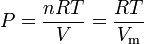# Molar gas constant

Values of R Units
8.314472 J·K-1·mol-1
0.082057 L·atm·K-1·mol-1
8.205745 × 10-5 m3·atm·K-1·mol-1
8.314472 L·kPa·K-1·mol-1
8.314472 m3·Pa·K-1·mol-1
62.36367 mmHg·K-1·mol-1
62.36367 Torr·K-1·mol-1
83.14472 L·mbar·K-1·mol-1
10.7316 ft3·psi· °R-1·lb-mol-1
0.73024 ft3·atm·°R-1·lb-mol-1

In chemistry, chemical engineering and physics, the molar gas constant (also called universal gas constant) R is a fundamental physical constant which appears in a large number of fundamental equations in the physical sciences, such as the ideal gas law and other equations of state and the Nernst equation. It is equivalent to the the Boltzmann constant (kB) times Avogadro's constant (N): R = kBNA.

Currently its most accurate value is:

R = 8.314472 J · K-1 · mol-1

The gas constant occurs in the ideal gas law as follows:$P = \frac{nRT}{V} = \frac{RT}{V_{\rm m}}$

where:

P is the gas absolute pressure
T is the gas absolute temperature
V is the volume the gas occupies
n is the number of moles of gas
Vm is the molar volume

##  Notation for the gas constant

The gas constant defined in this article is the universal gas constant, R, that applies to any gas. There is also a specific gas constant, which can be denoted as Rs. The specific gas constant is defined as Rs = R / M where M is the molecular weight.

Unfortunately, many authors in the technical literature sometimes use R as the specific gas constant without denoting it as such or stating that it is the specific gas constant. This can and does lead to confusion for many readers.

## Reference

1. Molar gas constant Obtained on 16 December, 2007 from the NIST websiteSome content on this page may previously have appeared on Citizendium.